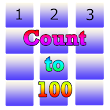Count to 100 Numbers for Kids APK

1.0 for Android
4.4/5The description of Count to 100 Numbers for Kids APK

Count to 100 Numbers for Kids is an important tool for helping kids learn to count to 100 and for helping kids learn to identify the numbers 0 through 100. Additionally, this tool enables users to understand the value of these numbers via the unit block depiction of each.

Count to 100 Numbers for Kids also provides an introduction to addition and subtraction. Clicking the +1 button adds 1 block to the display while the -1 button subtracts one.

The functionality of this app aligns to the following Common Core State Standards:

- CCSS.MATH.CONTENT.K.CC.A.1 - Count to 100 by ones and by tens.
- CCSS.MATH.CONTENT.K.CC.B.4.A - When counting objects, say the number names in the standard order, pairing each object with one and only one number name and each number name with one and only one object.
- CCSS.MATH.CONTENT.K.CC.B.4.B - Understand that the last number name said tells the number of objects counted. The number of objects is the same regardless of their arrangement or the order in which they were counted.
- CCSS.MATH.CONTENT.K.CC.B.4.C - Understand that each successive number name refers to a quantity that is one larger.